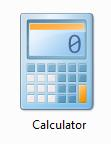# Smart Calculator

Smart Calculator allows you to easily create calculating forms for your web site.

Smart Calculator allows you to easily create calculating forms for your web site.

Features:

Variables:

Three type variable: integer, decimal and selection.

Set default value for a variable.

Set range for a variable.

Set view order for a variable.

Set title and help text for a variable.

Expression:

Support almost all math functions.

l         Operators such as +,-,*,/,^

l         Functions such as: abs, exp, log,log10, sign,sqrt

l         Trigonometry functions such as atan, sin, cos, tan.

l         Logical function IIF.

An expression can be a variable in another expression.

The module can check expressions to avoid circle calculating.

Set view order for an expression.

Set title and help text for an expression.

The result of expression can be numerical or text.

Set the format of calculating result.

Other fields:

Text: text or html.

Split: horizantal line to split fields.

Settings:

Set the css of title label.

Set the width of title label.

Set the css of calculating result.

Set the width of enter box.

Set the css of submit button.

Set the text of submit button.

Email notify:

Calculating result can be submitted to an email address that is defined by admin.

User can send the calculating report via email.

Report Template: admin can define the template of generated report.

Other features:

Import/Export: you can clone a calculator from one site to another easily.

Examples: there are several examples in the module.

Here are some examples of expression:

a * b

a * 3

exp(a)

log10(a)

a^b

sin(a)

IIF(a>10, “ Nice”, “ Fine'”)

## Standard Math Functions

 Math Function Evaluates: < Less than <> Not > Greater than + Add * Multiply () Math Brackets - Subtract ^ Power of / Divide

## Roots

To find the square root of a number you can use the Sqr function.

Sqrt(9) ' returns 3

One function that might not be quite so obvious is how to calculate the nth root of a number. To do this, all you need to do is use the following syntax:

nNumber ^ (1 / nRoot)

So, if you wanted to find the cubed root of 8 (ie root 3), all you need to do is

8 ^ (1 / 3)

and, if you wanted to find root 4 of 12, use

12 ^ (1 / 4)

## Absolute Value

The Abs function returns the absolute value of a number. This means that it returns a number that is always positive. For example,

Abs(12.3235)

Abs(-10.3)

## Numbers Sign

If you want to know if a number is positive or negative, use the Sign function. This will return -1 if the number is negative, and 1 if the number is positive. For example,

S i gn(-214)
S
ign(313.3)

## Exp

To get e raised to a power, call the Exp function:

Exp(1) ' returns e (2.71828182845905)
Exp(2) ' returns e to the power of 2 (7.38905609893065)

## Logarithms

To find the logarithm of a number, use the Log or Log10 function.

Log(1.31)

## Trigonometry Functions

The Trigonometry Functions in Visual Basic are fairly obvious, and are listed below.

 Trig. Function Description Atn Returns the arctangent of a number Acos Returns the arccosine of an angle Asin Returns the arcsine of an angle Cos Returns the cosine of an angle Sin Returns the sine of an angle Tan Returns the tangent of an angle

All these functions return the result as Double.